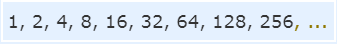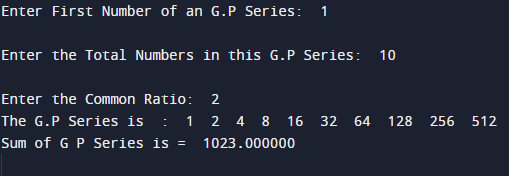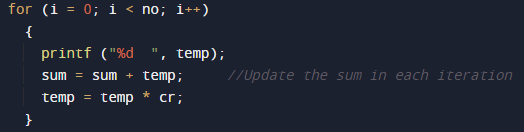# C Program To find Sum of GP Series

In this tutorial you will learn about the C Program To find Sum of GP Series and its application with practical example.

In this tutorial, we will learn to create a C program that will find Sum of GP using C programming.

## Prerequisites

Before starting with this tutorial we assume that you are best aware of the following C programming topics:

• Basic C programming.
• C Operators.
• Basic input/output.
• Loops.
• For loop.

## What Is geometric progression(GP)?

GP stands for geometric progression. It is defined as a Geometric Sequence of numbers each term is found by multiplying the previous term by a constant.

## ExampleThis sequence has a factor of 2.Each term (except the first term) is found by multiplying the previous term by 2.

Generally we write a Geometric Sequence like that:
{a, ar, ar2, ar3, … }
where:

• a is the first term, and
• r is the factor between the terms (called “common ratio”).

## C Program To find Sum of GP Series

In this program we will find sum of GP series using for loop. We would first declared and initialized the required variables. Next, we would prompt user to input the number of terms. Later we will generate the GP series of specified number of terms.

## OutputIn the above program, we have first declared and initialized a set variables required in the program.

• no = Total Numbers of terms to be printed
• f =  First term of series.
• cr= common ratio.
• sum= adding values of GP.
• temp=  holding temporary values.
• i= for iteration.

In the next statement user will be prompted to enter the number of terms which will be assigned to variable ‘no’ and first value in variable f and common ratio in cr.Now ,We will loop through the iterator ‘i’ up to the number of terms.Inside the for loop we are printing the value of temp variable and adding the sum of series in variable sum. When loop ends we print the sum of given gp. So in that way we find the sum of given gp number.

In this tutorial we have learn about the C Program To find Sum of GP Series and its application with practical example. I hope you will like this tutorial.